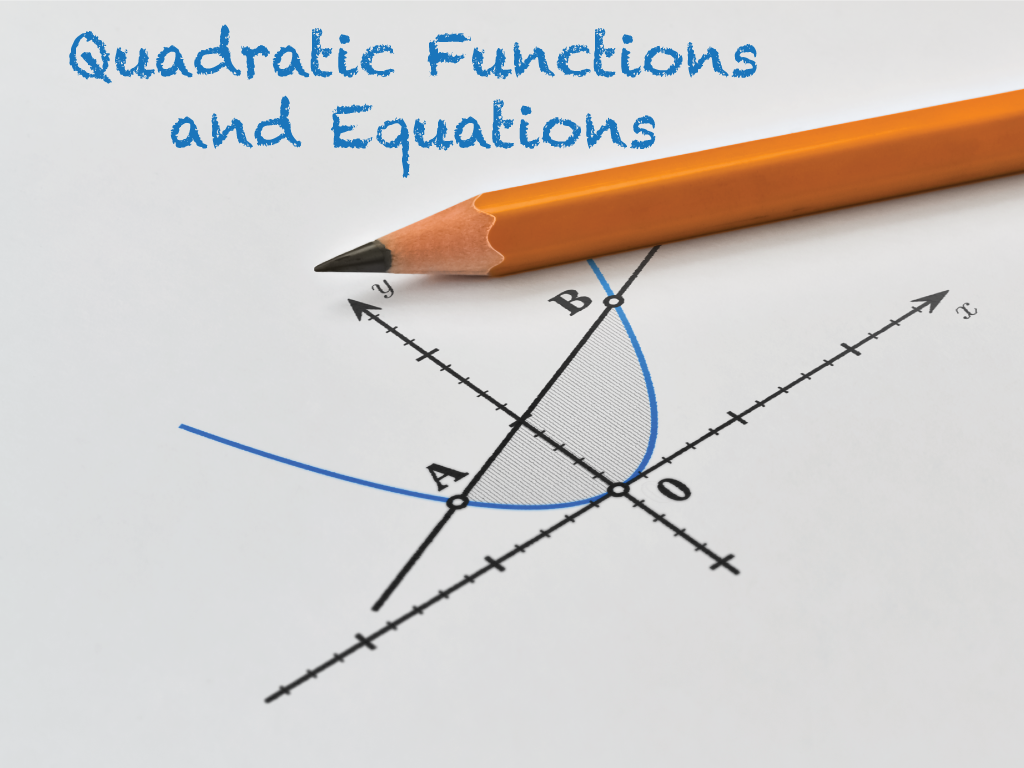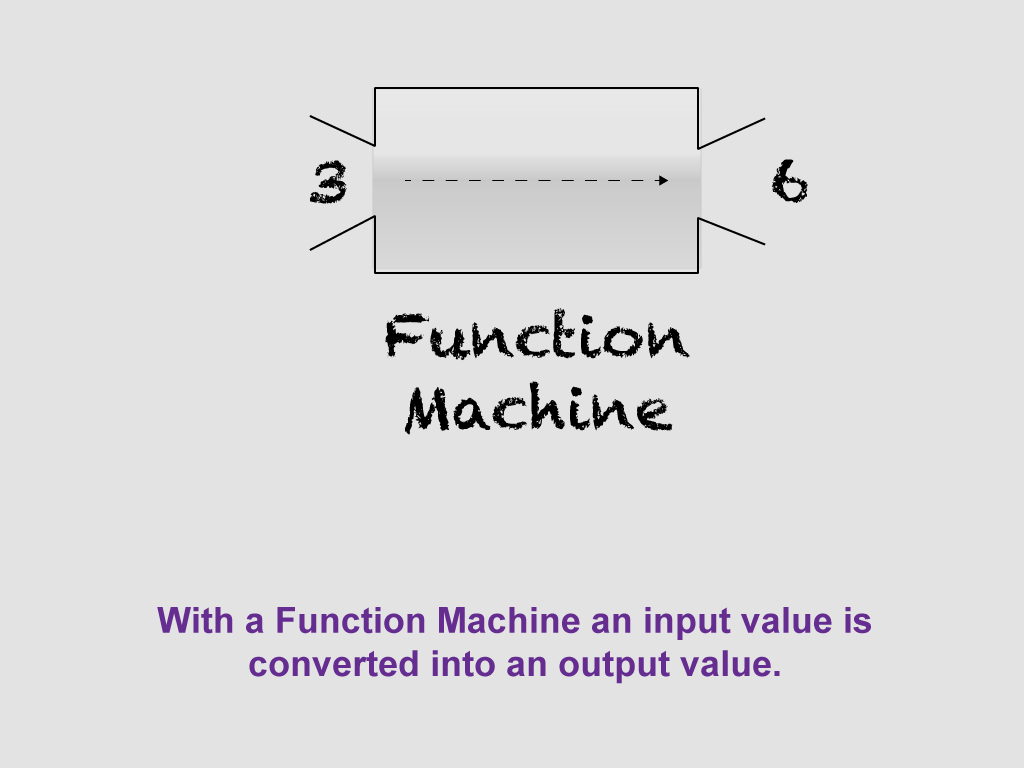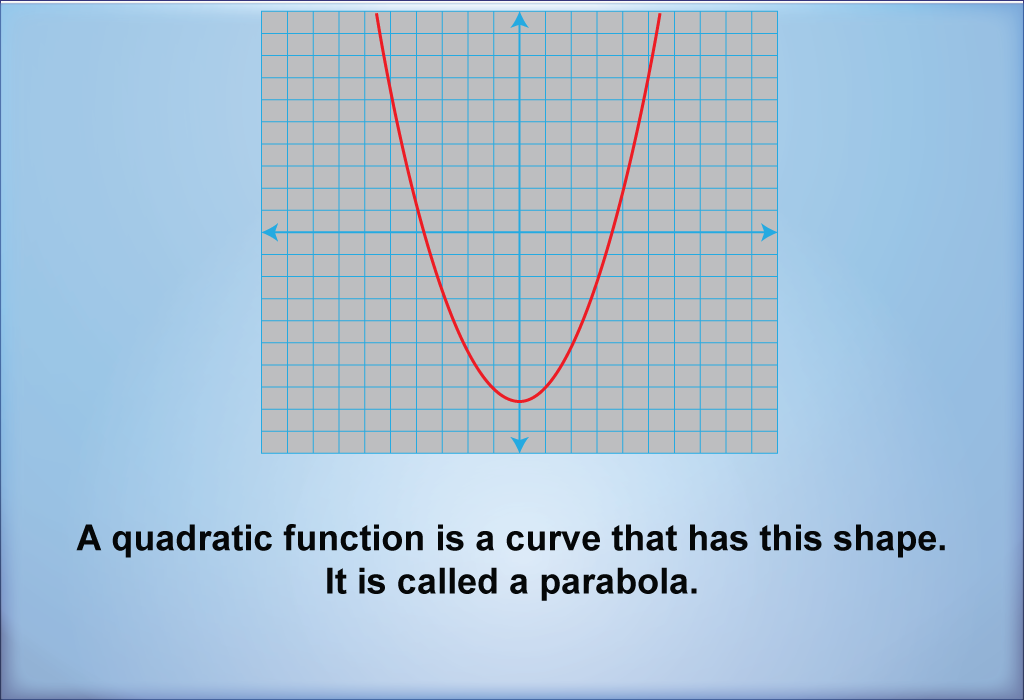# Topic Overview: Quadratic Functions and Equations## Overview

Before studying what a linear function is, make sure you are comfortable with the following concepts, which we will also review:

• What a function is
• Independent variable
• Dependent variable
• Domain
• Range
• Different representations of functions

## Brief Reviewof Functions

What Is a Function? A function is a one-to-one mapping of input values (the independent variable) to output values (the dependent variable). Click on this link to see a quick tutorial on what a function is. This slide show goes over the following key points:

• For every input value (x), there is a unique output value, f(x).
• Functions can be represented as equations, tables, and graphs.
• A function machine is a useful visual representation of the input/output nature of functions.Dependent/Independent Variables. When one variable depends on another, then it is the dependent variable. For example, the faster your speed, the farther you travel. Suppose that speed is represented by the variable s and the distance traveled is represented by the variable d

Here’s how to describe the relationship between s and d:

The faster the speed, the more distance traveled.

Distance is dependent on speed.

Distance is a function of speed.

d = f(s)

When studying functions, make sure you are comfortable telling the difference between the independent variable and dependent variable. Get comfortable using function notation. To learn more about function notation, click on this link.

Domain and Range. A function shows the relationship between two variables, the independent variable and the dependent variable. The domain is the allowed values for the independent variable. The range is the allowed values for the dependent variable. The domain and range influence what the graph of the function looks like.

For a detailed review of what domain and range are, click on this link to learn more. You’ll see definitions of the terms domain and range, as well as examples of how to find the domain and range for given functions.

Multiple Representations of Functions. We mentioned previously that functions can be represented in different ways. In fact, any function can be represented by an equation, usually f(x) equal to some expression; a table; or a graph. For a detailed review of multiple representations of functions, click on this link, to see a slide show that includes examples of these multiple representations.

### Standard Form

A quadratic function in standard form has an equation that looks like this:In this form, the parameters a, b, and c are real numbers and a can’t be equal to zero. The term c is also the y-intercept of the function.

### Vertex Form

A quadratic function in vertex form has an equation that looks like this:In this form, the parameter a is identical to the same parameter in the standard form. The parameters h and k are and the x- and y-coordinates, respectively, for the coordinates of the vertex of the quadratic function.

The graph of a quadratic function is called a parabolaFor a detailed overview of quadratic functions in standard and vertex form, click here to view this slide show.

A quadratic equation in standard form is of this form:A quadratic equation can have two, one, or zero real number solutions. To find the solutions to a quadratic equation, use the quadratic formulaBefore using the quadratic formula, calculate the discriminant:Here’s how to interpret values for the discriminant (d):

 Value of d Number of Real Number Solutions d > 0 Two solutions d = 0 One solution d < 0 No solutions

For a detailed overview of solving quadratic equations click on this link to see a slide show. This will include several videos and detailed math examples.